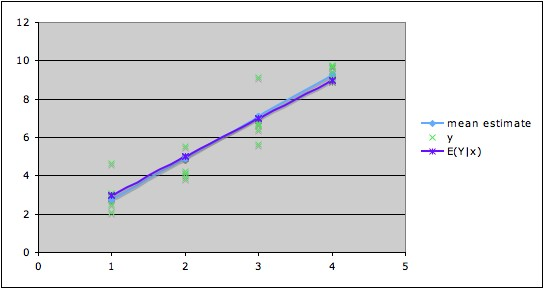## This site is under construction. Please check back every few weeks for updates

### COMMON MISTEAKS MISTAKES IN USING STATISTICS: Spotting and Avoiding Them

Interpreting a Coefficient as a Rate of Change in Y Instead of as a Rate of Change in the Conditional Mean of Y.

As pointed out in the discussion of overfitting, the computed regression equation estimates the true conditional mean function. How well it estimates the behavior of actual values of the random variable depends on the variability of the response variable Y. Thus, interpreting the computed coefficients in terms of the response variable is often misleading.

Illustration: In the graph shown below, the data are marked in green, the true line of conditional means is in violet, and the  fitted (
computed) regression line is in blue. Note that the fitted regression line is close to the true line of conditional means. The equation of the fitted regression line is (with coefficients rounded to a reasonable degree) ŷ = 0.56 + 2.18x.1 Thus it is accurate to say, "For each change of one unit in x, the average change in the mean of Y is about 2.18 units." It is not accurate to say, "For each change of one unit in x, Y changes about 2.18 units." For example, we can see from the graph that when x is 2, Y might be anywhere between a little below 4 to a little above 5.5; when x is 3, Y might be anywhere from a little more than 5.5 to a little more than 9. So when going from x = 2 to x = 3, the change in Y might be almost zero, or it might be as large as 5.5 units.Notes:
1. The true line of means in this constructed example is E(Y|X = x) = 1 + 2x.